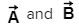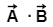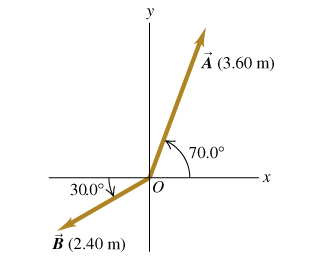# Problem: 1) For the two vectors  in the figure (Figure 1), find the scalar product Express your answer in square meters.

###### FREE Expert Solution

Vector components:

From vector components equation:

Ax = (3.60) cos (70°) = 1.23m i

Ay = (3.60) sin (70°) = 3.38m j

A = (1.23 i + 3.38 j) m

100% (204 ratings)###### Problem Details

1) For the two vectorsin the figure (Figure 1), find the scalar product